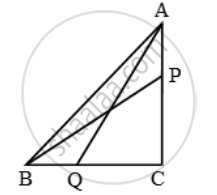# In a Right Triangle Abc Right-angled at C, P and Q Are the Points on the Sides Ca and Cb Respectively, Which Divide These Sides in the Ratio 2 : 1. Prove that - Mathematics

Sum

In a right triangle ABC right-angled at C, P and Q are the points on the sides CA and CB respectively, which divide these sides in the ratio 2 : 1. Prove that

(i) 9 AQ^2 = 9 AC^2 + 4 BC^2

(ii) 9 BP^2 = 9 BC^2 + 4 AC^2

(iii) 9 (AQ^2 + BP^2 ) = 13 AB^2

#### Solution

It is given that P divides CA in the ratio 2 : 1. Therefore,

CP=\frac { 2 }{ 3 }AC ….(i)

Also, Q divides CB in the ratio 2 : 1.

∴ QC=\frac { 2 }{ 3 }BC ….(ii)(i) Applying pythagoras theorem in right-angled triangle ACQ, we have

AQ^2 = QC^2 + AC^2

⇒ AQ^2 = \frac { 4 }{ 9 } BC^2 + AC^2

⇒ 9 AQ^2 = 4 BC^2 + 9 AC^2 ….(iii)

(ii)Applying pythagoras theorem in right triangle BCP, we have

BP^2 = BC^2 + CP^2

⇒ BP^2 = BC^2 + AC^2

⇒ 9 BP^2 = 9 BC^2 + 4 AC^2 ….(iv)

(iii)Adding (iii) and (iv), we get

9 (AQ^2 + BP^2 ) = 13 (BC^2 + AC^2 )

⇒ 9 (AQ^2 + BP^2 ) = 13 AB^2 [∵ BC^2 = AC^2 + AB^2 ]

Is there an error in this question or solution?

Share